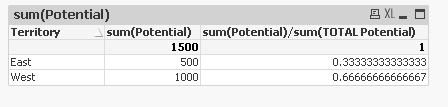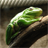# QlikView App Dev

Discussion Board for collaboration related to QlikView App Development.

Announcements
Welcome to Qlik Community! Check out our new navigation! FIND OUT MORE
cancel
Showing results for
Did you mean:Not applicable

## Divide Field by Total of the Whole Column

TerritoryPotentialBPI
East500Potential / Total Potential or 500/1500
West1000
Total Potential1500

I want to take the Potential for each territory and divide it by the Potential of ALL Territories, but cannot get this to work.  I was trying set analysis (and we use Max(Year) in our app to accommodate the user selection).

I tried this:

(Sum({\$<Year={\$(=Max(Year))}>} [Sales Potential])) /  (Sum({\$<Year={\$(=Max(Year))}, [Territory]=>} [Sales Potential]))

But it doesn't work.  It appears to be ignoring the removal of the Territory in the formula as the calculation = 1 in every instance.  So, it's dividing Territory Potential by Territory Potential instead of Territory Potential / Total Potential.

1 Solution

Accepted SolutionsSpecialist

so the first option should work. sum(Potential)/sum(TOTAL Potential)...here "TOTAL" is a key word.10 RepliesSpecialist II

Try something like:

sum(Potential)/sum(total Potential)

Hope this helps!MVP

SUM(Potential)/SUM(Total Potential)

Or

SUM(Potential)/SUM({1}Potential)

or

SUM(Potential)/SUM(All Potential)Specialist

assuming Total Potential is not an actual value in the Territory field, what happens if you do sum(Potential)/sum(Total Potential).

if Total Potential is an actual value in Territory field, you can use set analysis, something like:

sum(Potential)/sum({<Territory='Total Potential'>} Potential)

you can use your other set expressions (for max(Year), etc.) in both options:

sum(Potential)/sum({<Year={'max(Year)'} total Potential)

or

sum(Potential)/sum({<Territory={'Total Potential'}, Year={'max(Year)'}>} Potential)Not applicable
Author

Thanks, but the Total Potential is just the total of the expression as calculated by QV. So, it’s not a distinct value.

There are multiple columns in this table and dividing by Total Potential seems to be unrecognized by QV. Total Potential is not really a distinct field.

Thanks,

ShelleyPartner - Creator II

Hi,

you could also just check "Relative" for this Expression which is similar to sum(Total Value). It goes only by the total of the selction. To make it go by the total of all no matter what is selected you hav to use sum(all Value).

Check my demo and do selections.

Hope this helps.

TobiasPartner - Specialist

HI,

You can use sum(Potential) \ sum( TOTAL Potential)

-SathishSpecialist

so the first option should work. sum(Potential)/sum(TOTAL Potential)...here "TOTAL" is a key word.Not applicable
Author

Okay!  Thank you for pointing out that TOTAL is a key word.  I finally got it to work.  Thanks so much!Not applicable
Author

THIS WORKS TOO AND IS VERY EASY!!!  THANKS FOR YOUR HELP!